# Model Diagnostics - Teradata Warehouse Miner

## Teradata Warehouse Miner User Guide - Volume 3Analytic Functions

Product
Release Number
5.4.5
Published
February 2018
Language
English (United States)
Last Update
2018-05-04
dita:mapPath
yuy1504291362546.ditamap
dita:ditavalPath
ft:empty
dita:id
tbj1499730075274
Product Category
Software

One of the advantages in using a statistical modeling technique such as linear regression (as opposed to a machine learning technique, for example) is the ability to compute rigorous, well-understood measurements of the effectiveness of the model. Most of these measurements are based upon a huge body of work in the areas of probability and probability theory.

## Goodness of fit

Several model diagnostics are provided to give an assessment of the effectiveness of the overall model. One of these is called the residual sums of squares or sum of squared errors RSS, which is simply the sum of the squared differences between the dependent variable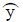estimated by the model and the actual value of y, over all of the rows: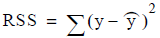Now suppose a similar measure was created based on a naive estimate of y, namely the mean value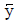: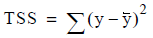often called the total sums of squares about the mean.

Then, a measure of the improvement of the fit given by the linear regression model is given by: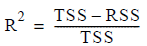This is called the squared multiple correlation coefficient R2, which has a value between 0 and 1, with 1 indicating the maximum improvement in fit over estimating y naively with the mean value of y. The multiple correlation coefficient R is actually the correlation between the real y values and the values predicted based on the independent x variables, sometimes written Ry · x1x2...xn , which is calculated here simply as the positive square root of the R2 value. A variation of this measure adjusted for the number of observations and independent variables in the model is given by the adjusted R2 value: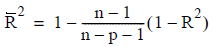where n is the number of observations and p is the number of independent variables (substitute n-p in the denominator if there is no constant term).

The numerator in the equation for R2, namely TSS - RSS, is sometimes called the due-to-regression sums of squares or DRS. Another way of looking at this is that the total unexplained variation about the mean TSS is equal to the variation due to regression DRS plus the unexplained residual variation RSS. This leads to an equation sometimes known as the fundamental equation of regression analysis: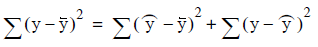Which is the same as saying that TSS = DRS + RSS. From these values a statistical test called an F-test can be made to determine if all the x variables taken together explain a significant amount of variation in y. This test is carried out on the F-ratio given by: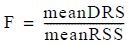The values meanDRS and meanRSS are calculated by dividing DRS and RSS by their respective degrees of freedom (p for DRS and n-p-1 for RSS).

## Standard errors and confidence intervals

Measurements are made of the standard deviation of the sampling distribution of each b-coefficient value, and from this, estimates of a confidence interval for each of the coefficients are made. For example, if one of the coefficients has a value of 6, and a 95% confidence interval of 5 to 7, it can be said that the true population coefficient is contained in this interval, with a confidence coefficient of 95%. In other words, if repeated samples were taken of the same size from the population, then 95% of the intervals like the one constructed here, would contain the true value for the population coefficient.

Another set of useful statistics is calculated as the ratio of each b-coefficient value to its standard error. This statistic is sometimes called a T-statistic or Wald statistic. Along with its associated t-distribution probability value, it can be used to assess the statistical significance of this term in the model.

## Standardized coefficients

The least-squares estimates of the b-coefficients are converted to so-called beta-coefficients or standardized coefficients to give a model in terms of the z-scores of the independent variables. That is, the entire model is recast to use standardized values of the variables and the coefficients are recomputed accordingly. Standardized values cast each variable into units measuring the number of standard deviations away from the mean value for that variable. The advantage of doing this is that the values of the coefficients are scaled equivalently so that their relative importance in the model can be more easily seen. Otherwise the coefficient for a variable such as income would be difficult to compare to a variable such as age or the number of years an account has been open.

## Incremental R-squared

It is possible to calculate the value R2 incrementally by considering the cumulative contributions of x variables added to the model one at a time, namely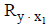,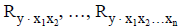. These are called incremental R2 values, and they give a measure of how much the addition of each x variable contributes to explaining the variation in y in the observations. This points out the fact that the order in which the independent x variables are specified in creating the model is important.

## Multiple Correlation Coefficients

Another measure that can be computed for each independent variable in the model is the squared multiple correlation coefficient with respect to the other independent variables in the model taken together. These values range from 0 to1 with 0 indicating a lack of correlation and 1 indicating the maximum correlation.

Multiple correlation coefficients are sometimes presented in related forms such as variance inflation factors or tolerances. A variance inflation factor is given by the formula: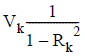Where Vk is the variance inflation factor and Rk 2 is the squared multiple correlation coefficient for the k th independent variable. Tolerance is given by the formula T k = 1 - R k 2, where T k is the tolerance of the k th independent variable and Rk 2 is as before.

These values may be of limited value as indicators of possible collinearity or near dependencies among variables in the case of high correlation values, but the absence of high correlation values does not necessarily indicate the absence of collinearity problems. Further, multiple correlation coefficients are unable to distinguish between several near dependencies should they exist. The reader is referred to [Belsley, Kuh and Welsch] for more information on collinearity diagnostics, as well as to the upcoming section on the subject.